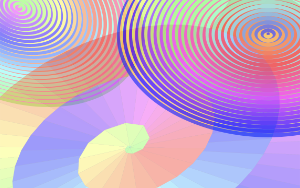# Snail of Theodorus

## A variation on the Archimedean Spiral...## First the Maths...

The animation at the bottom of the page came about as a direct result of reading Robert Ferréol’s page about the Archimedean spiral. The page shows how the Archimedean spiral follows a string of right angled triangles where the hypoteneuse side of one becomes the adjacent side of the next triangle (working from the spiral's centre angle), starting with an isosceles right angled triangle.

You can use Pythagoras’ theorem to calculate the path of the spiral created (see the diagram below). Knowing that the square and the square root of 1 are both 1 then,assuming the lenth of the two equal sides 1, then 12 plus 12 equals two, making the length of the hypoteneuse (the other side) must be √2. Extending the spiral going out from there then the length of each hypoteneuse is √3, √4, √5 and so on...

I then looked for a way to extend the spiral inwards from the initial isosceles triange, and what I came up with (outlined in dotted lines below) was more isosceles right anged triangles where the adjacenet of one becomes the hypoteneuse of the next. Here, if the hypoteneuse is 1 then both the opposite and adjacent will equal √0.5. Contextually not part of the Snail of Theodurus, but it works visually so I ran with it.## ...and now, the animationTo create the animation I used and modified the styles from the other animation. The first thing I did was to remove the rotation of the image. I sped up the rate at which the colours change and reduced the opacity of the image, making the colours lighter.

I then set the colour styles in each of the triangles so as to make the colours appear to move in from the outside and out from the centre along the spiral. They then meet somewhere near half way, just off centre at the bottom of the image.

This animation took a lot less time as I'd already done most of the coding, leaving me the drawing and a bit of code editing.

## The End